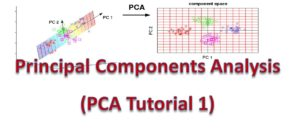# PCA Tutorial 1 – Introduction to PCA and Dimensionality Reduction

PCA is one of the concepts that many find a bit tough to grasp. I had the same issue, but now I figure out a way to explain it in simple and clear term. But we actually need to start from the concept of dimensionality reduction.This tutorial assumes you don’t have any knowledge of Principal Components Analysis. But it is expected that you have some basic knowledge of matrix operation

We are going to cover the following areas

#### 1. Introduction to Dimensionality Reduction

Dimensionality refers to the number of features associated with each data measurement. It corresponds to the columns in an tabular data.

When a dataset is beyond 2d or 3d then it would be difficult to visualize.  But most datasets used for analysis are in very large dimension, say tens or even hundreds. So how do we manage this scenario. That is where the concept of dimensionality reduction comes into play.

Dimensionality reduction in statistics and  machine learning is the process by which the number of random variables under consideration is reduced by obtaining a set of few principal variables.

#### 2. Problem with  High-Dimensional Data

Let’s now outline the four problems with high-dimensional data that makes dimensionality reduction very important

a) Time and Space Complexity: Training of a model with a very high-dimensional data incurs high space and time complexity. This simply means that more memory space is required and much processing time is needed as well

b) Problem of Over-fitting: High-dimensional data may also lead to problem of over-fitting where the model created is not able to capture completely new data points

c) Redundant Features: Not all the features of the data is relevant

d) Noise: Data in lower dimension generally have lower noise (or unnecessary data)

#### 3. Types of Dimensionality Reduction

It is necessary for you to know that that there are two types of dimensionality reduction, namely:

Feature Extraction: This is technique that has to to with finding new features in the data after it has been transformed form high-dimensional data to low dimensional space.

Feature Selection: The is the technique of finding the most relevant features of a given data. It is done by obtaining a subset of key feature of the variables in the original dataset.

The difference is that while feature extraction is interested in finding new variables, feature selection focuses on selecting most relevant features from existing data.

#### 4.  Methods of Dimensionality Reduction

Although we would be discussing Principal Components Analysis in this blog, I would also like to let you know the various other algorithms that exist for dimensionality reduction. We would just highlight four method

a) Principal Components Analysis(PCA): The method applies linear approximation to find out the components that contribute most to the variance in the dataset.

b) Multidimensional Scaling (MDS): This is a dimensionality reduction technique that works by creating a map  of relative positions of data points in the dataset.

c) Factor Analysis(FA):  This is a statistical method that is used to describe the variation existing among observed and correlated variables in terms of unobserved variables which are fewer in number.

d) Independent Components Analysis(ICA): This is technique that begins with factor analysis and searches of rotations in the data that leads to independent components.

Let’s now focus on Principal Components Analysis (PCA)

#### 5. What is Principal Components Analysis(PCA)

There a many ways we can define PCA. But for now, let’s use a simple definition. PCA is a variance-maximizing technique that projects the existing data onto a direction that maximizes variance. PCA performs a linear mapping of the original data onto a lower-dimensional sub-space such that the variability of the data in low-dimension is maximized. We would simply this is a  minute.

PCA is an unsupervised learning approach since it uses only a set of features X1, X2, . . ., Xp without any classes or labels. PCA is a process of calculating the principal components and using it to explain the data.

#### 6. What Really are Principal Components.

Let’s start with a dataset of n observations and p features where p is very large. The notion is that each of the n observations is in p-dimensional space. Therefore for each observation, not all of the dimensions are very useful. We are interesting in finding out the amount of each observation that vary along each dimension.

Each of the dimension that is discovered by PCA is said to be a linear combination of the p features. We would now discuss the details of finding the Principal Components in PCA Tutorial 2.#### kindsonthegenius

Kindson Munonye is currently completing his doctoral program in Software Engineering in Budapest University of Technology and Economics

View all posts by kindsonthegenius →

## 6 thoughts on “PCA Tutorial 1 – Introduction to PCA and Dimensionality Reduction”

1.kindsonthegenius says:

This is a test Comment

2. Pingback: 20 Cool Machine Learning and Data Science Concepts (Simple Definitions) - The Genius Blog